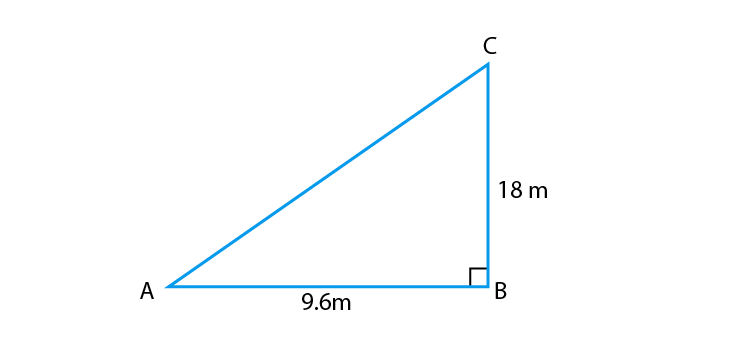# A flag pole 18 m high casts a shadow 9.6 m long. Find the distance of the top of the pole from the far end of the shadow.Solution

Let MN = 18 m be the flag pole and its shadow be LM = 9.6 m.

The distance of the top of the pole, N from the far end, L of the shadow is LN.

From right-angled ∆LMN,

LN2 = LM2 + MN2

On applying Pythagoras theorem we obtain

⇒ LN2 = (9.6)2 + (18)2

⇒ LN2 = 9.216 + 324

⇒ LN2 = 416.16

∴ LN = √416.16 = 20.4 m

Hence, the required distance is 20.4 m(0)(0)